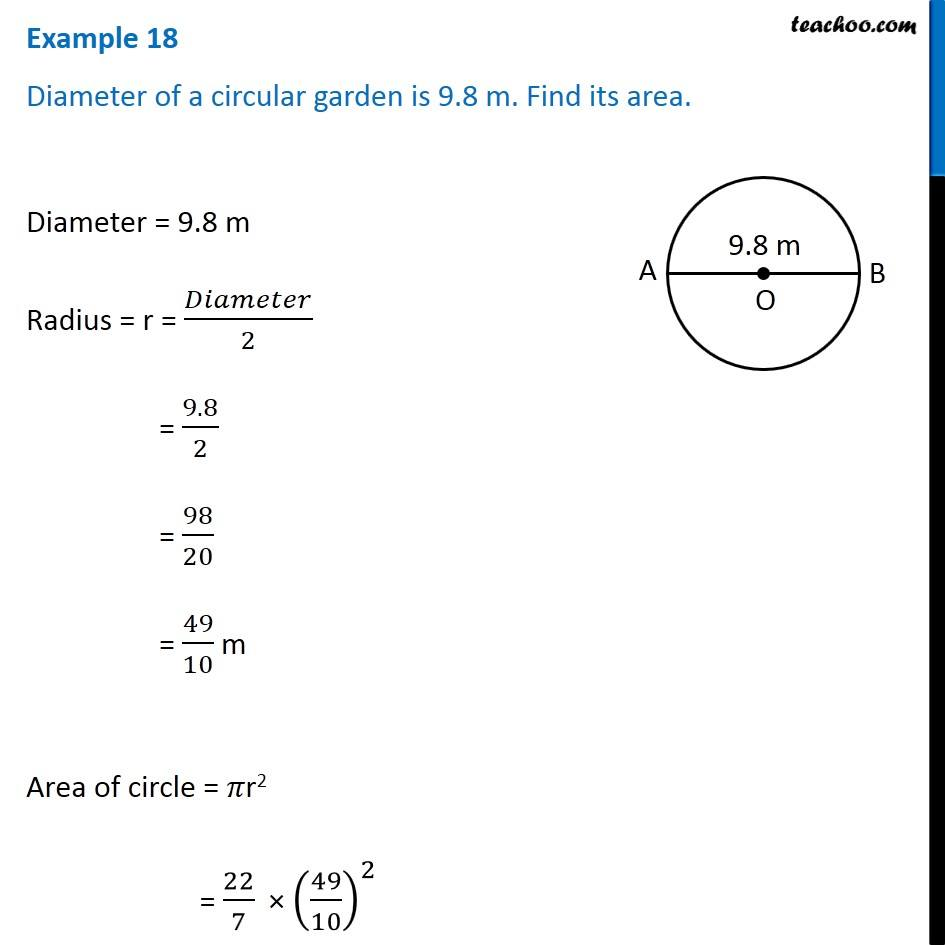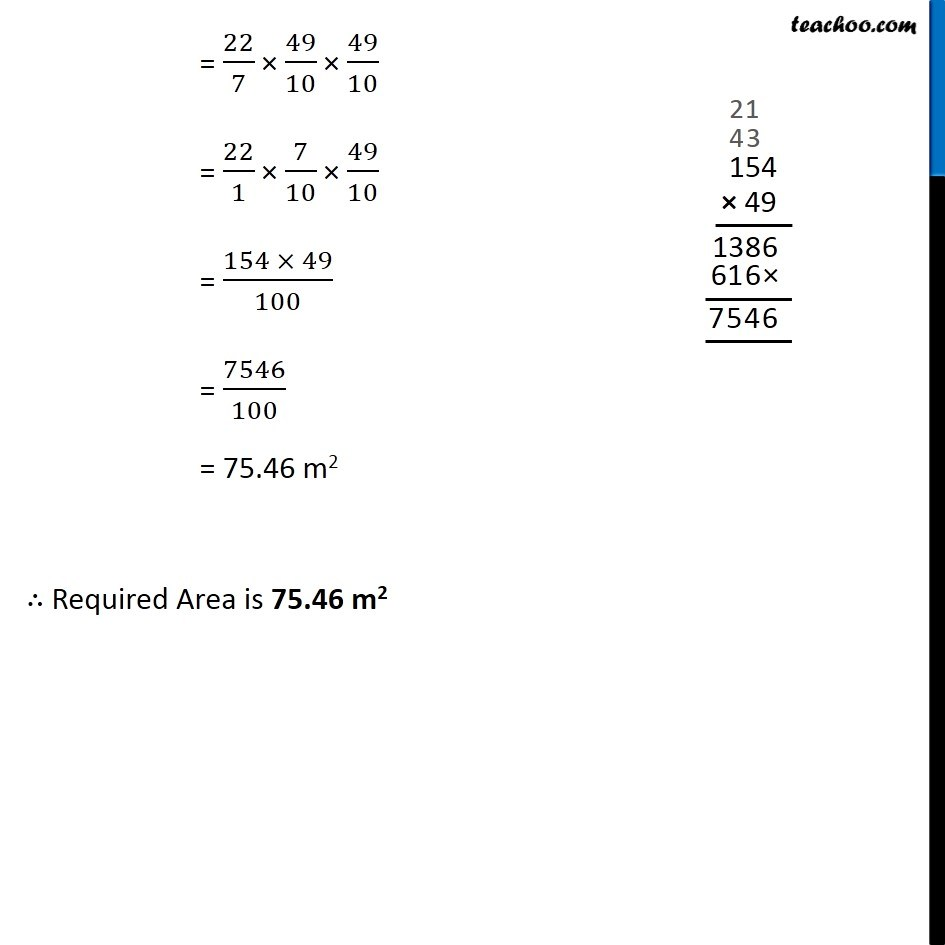1. Chapter 11 Class 7 Perimeter and Area
2. Serial order wise
3. Examples

Transcript

Example 18 Diameter of a circular garden is 9.8 m. Find its area. Diameter = 9.8 m Radius = r = 𝐷𝑖𝑎𝑚𝑒𝑡𝑒𝑟/2 = 9.8/2 = 98/20 = 49/10 m Area of circle = 𝜋r2 = 22/7 × (49/10)^2 = 22/7 × 49/10 × 49/10 = 22/1 × 7/10 × 49/10 = (154 × 49)/100 = 7546/100 = 75.46 m2 ∴ Required Area is 75.46 m2

Examples

Chapter 11 Class 7 Perimeter and Area
Serial order wise